# Introduction

etree is the R package where Energy Trees (Giubilei et al., 2022) are implemented. The model allows to perform classification and regression with covariates that are possibly structured and of different types.

This vignette includes two examples, one for classification and one for regression, where all the package’s basic functionalities are explained. The variables’ types included in the examples are those currently available in the package: numeric, nominal, functional, and in the form of graphs.

The first thing to do to run the examples is loading the package etree.

library(etree)

# Data generation

We need data for our examples. We can use the same covariates for regression and classification, and then change only the response. Let us consider $$100$$ observations divided into four equal-sized and disjoint groups $$G_1, G_2, G_3, G_4$$. The response variable for regression is defined as:

$Y^R_i \sim \begin{cases} \mathcal{N}(0, 1) & \text{for} \; i \in G_1\\ \mathcal{N}(1, 1) & \text{for} \; i \in G_2\\ \mathcal{N}(2, 1) & \text{for} \; i \in G_3\\ \mathcal{N}(3, 1) & \text{for} \; i \in G_4 \end{cases}.$

The corresponding R object must be of class “numeric” to be correctly recognized by etree() as a response variable for regression.

# Set seed
set.seed(123)

# Number of observation
n_obs <- 100

# Response variable for regression
resp_reg <- c(rnorm(n_obs / 4, mean = 0, sd = 1),
rnorm(n_obs / 4, mean = 2, sd = 1),
rnorm(n_obs / 4, mean = 4, sd = 1),
rnorm(n_obs / 4, mean = 6, sd = 1))

The response for classification is a nominal variable measured at four different levels: $$1, 2, 3, 4$$. For each group, observations are randomly assigned one of the four levels. The probability distribution is different for each group, and it gives the largest probability to the level equal to the index of the group, uniformly allocating the rest to the other three levels. For example, the response variable for the first group is the following:

$Y^C_i \sim \begin{cases} 1 & p = 0.85 \\ 2 & p = 0.05 \\ 3 & p = 0.05 \\ 4 & p = 0.05 \end{cases}, \quad \text{for} \; i \in G_1.$

The same holds for $$G_2, G_3, G_4$$, except for switching each time the probabilities in such a way that the most probable level matches the index of the group.

The response variable for classification must be defined as a factor to be correctly identified by etree().

# Response variable for classification
resp_cls <- factor(c(sample(x = 1:4, size = n_obs / 4, replace = TRUE,
prob = c(0.85, 0.05, 0.05, 0.05)),
sample(x = 1:4, size = n_obs / 4, replace = TRUE,
prob = c(0.05, 0.85, 0.05, 0.05)),
sample(x = 1:4, size = n_obs / 4, replace = TRUE,
prob = c(0.05, 0.05, 0.85, 0.05)),
sample(x = 1:4, size = n_obs / 4, replace = TRUE,
prob = c(0.05, 0.05, 0.05, 0.85))))

For both tasks, covariates are defined as follows:

1. Numeric: $X_{1i} \sim \begin{cases} \mathcal{U}[0, 0.55] & \text{for} \; i \in G_1\\ \mathcal{U}[0.45, 1] & \text{for} \; i \in G_2 \cup G_3 \cup G_4 \end{cases};$

2. Nominal: $X_{2i} \sim \begin{cases} \text{Bern}(0.1) & \text{for} \; i \in G_2\\ \text{Bern}(0.9) & \text{for} \; i \in G_1 \cup G_3 \cup G_4 \end{cases};$

3. Functions: $X_{3i} \sim \begin{cases} \mathcal{GP}(0, I_{100}) & \text{for} \; \in G_3\\ \mathcal{GP}(1, I_{100}) & \text{for} \; \in G_1 \cup G_2 \cup G_4 \end{cases},$

where $$\mathcal{GP}$$ is a Gaussian random process over $$100$$ evaluation points from $$0$$ to $$1$$;

1. Graphs: $X_{4i} \sim \begin{cases} \text{ER}(100, 0.1) & \text{for} \; \in G_4\\ \text{ER}(100, 0.9) & \text{for} \; \in G_1 \cup G_2 \cup G_3 \end{cases},$

where $$\text{ER}(n, p)$$ is an Erdős–Rényi random graph with $$n$$ vertices and $$p$$ as connection probability.

The structure of the generative process is such that we need at least three splits with respect to three different covariates to correctly rebuild the four groups.

The set of covariates must be generated as a list, where each element is a different variable. Additionally, numeric variables must be numeric vectors, nominal variable must be factors, functional variables must be objects of class “fdata”, and variables in the forms of graphs must be lists of objects having class “igraph”.

# Numeric
x1 <- c(runif(n_obs / 4, min = 0, max = 0.55),
runif(n_obs / 4 * 3, min = 0.45, max = 1))

# Nominal
x2 <- factor(c(rbinom(n_obs / 4, 1, 0.1),
rbinom(n_obs / 4, 1, 0.9),
rbinom(n_obs / 2, 1, 0.1)))

# Functions
x3 <- c(fda.usc::rproc2fdata(n_obs / 2, seq(0, 1, len = 100), sigma = 1),
fda.usc::rproc2fdata(n_obs / 4, seq(0, 1, len = 100), sigma = 1,
mu = rep(1, 100)),
fda.usc::rproc2fdata(n_obs / 4, seq(0, 1, len = 100), sigma = 1))

# Graphs
x4 <- c(lapply(1:(n_obs / 4 * 3), function(j) igraph::sample_gnp(100, 0.1)),
lapply(1:(n_obs / 4), function(j) igraph::sample_gnp(100, 0.9)))

# Covariates list
cov_list <- list(X1 = x1, X2 = x2, X3 = x3, X4 = x4)

# Regression

The first example is regression. The set of covariates is cov_list and the response variable is resp_reg. We start with a “quick fit”, which implies using etree()’s default parameters. Then, we explore how changing the most important ones affects the size and the structure of the resulting trees. Finally, we use a fitted tree to explore other fundamental utilities such as printing and predicting.

## Quick fit

The quickest way to grow an Energy Tree is as simple as using etree() and specifying the response and the set of covariates only.

# Quick fit
etree_fit <- etree(response = resp_reg,
covariates = cov_list)

Calling plot() on the fitted object etree_fit allows visualizing the tree.

# Plot
plot(etree_fit)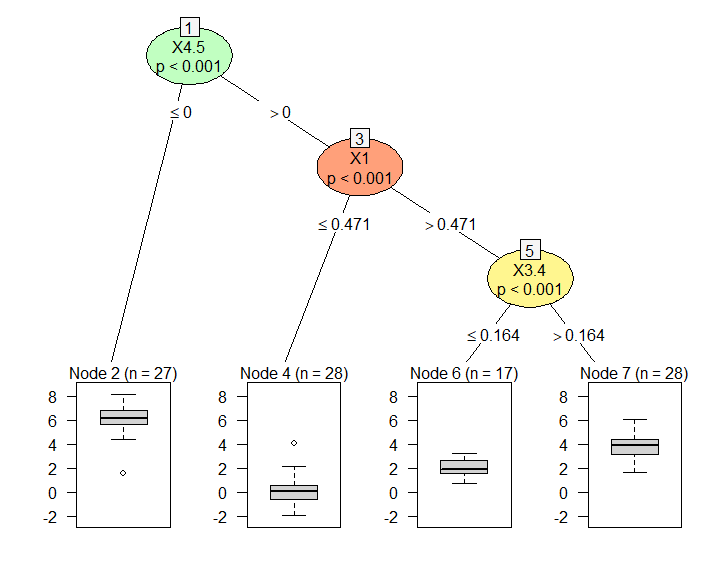This is a good place to stop and comment the main features of etree’s plots. Many of them descend from the partykit (Hothorn and Zeileis, 2015) version:

• the number in the square box is the id of the node;
• the text below the square box is the name of the variable selected for splitting;
• the number right below is the p-value for the Energy test of independence between the splitting covariate and the response variable;
• when the splitting covariate is traditional, values on the edges represent the split point for that variable;
• terminal nodes have a header showing the id and the size of the node;
• terminal nodes contain different plot types, which depend on the nature of the response: bar plots for classification, box plots for regression.

In the etree version, some novelties have been introduced:

• inner nodes are colored differently to distinguish the various types of covariates: salmon for numeric variables, light blue for nominal variables, yellow for functions, light green for variables in the form of graphs;
• when the splitting covariate is structured and split_type = “coeff”, the text below the square box is the name of the splitting covariate followed by a dot and the id of the selected component;
• when the splitting covariate is structured, values on the edges represent the split point with respect to the selected component of the splitting covariate when split_type = “coeff”, or alternatively the size of the corresponding kid node when split_type = “cluster”.

In the specific fit, not only the partitioning into four groups is correctly retrieved, but also the average response variable in each group is consistent with the mean of the distributions used to generate data.

Now we explore how the main optional parameters work and their effects on the structure and size of the resulting trees.

## Changing parameters

The two parameters directly influencing the tree size are minbucket and alpha.

The first one, minbucket, sets the minimum number of observations each node must contain. When it grows, the tree gets smaller; and viceversa. Let’s see what happens if we set it equal to its minimum, i.e., 1.

# Fit with smaller minbucket
etree_fit1 <- etree(response = resp_reg,
covariates = cov_list,
minbucket = 1)

# Plot
plot(etree_fit1)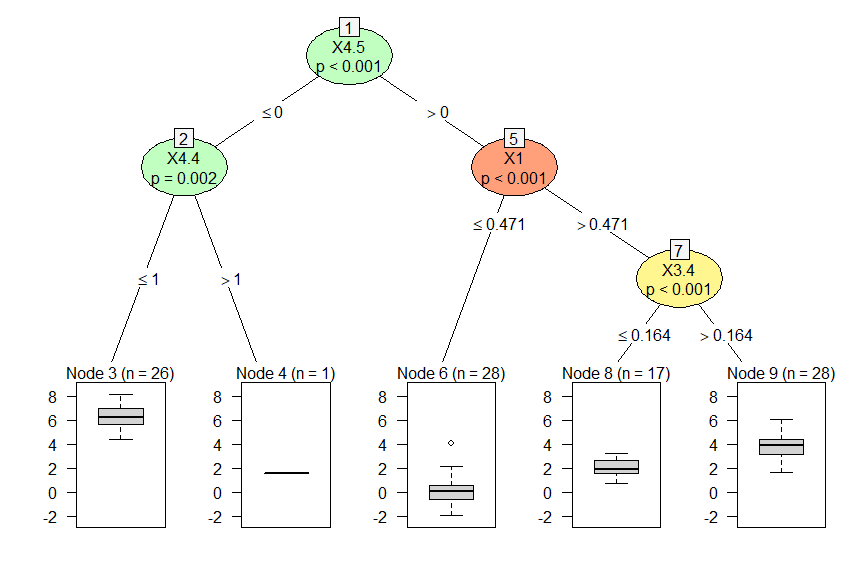We actually get a larger tree with five terminal nodes. The additional one derives from the split of node 2 into two nodes with size 26 and 1, respectively. Increasing minbucket to any value would result in avoiding that split.

The other parameter controlling the tree size is alpha, which is the significance level in the Energy tests of independence used for variable selection. When alpha decreases, we can expect that the tree gets smaller, because we would only consider stronger associations between the covariates and the response; and viceversa.

We can increase alpha to its maximum, which is 1, to see what happens.

# Fit with larger alpha
etree_fit2 <- etree(response = resp_reg,
covariates = cov_list,
alpha = 1)

# Plot
plot(etree_fit2)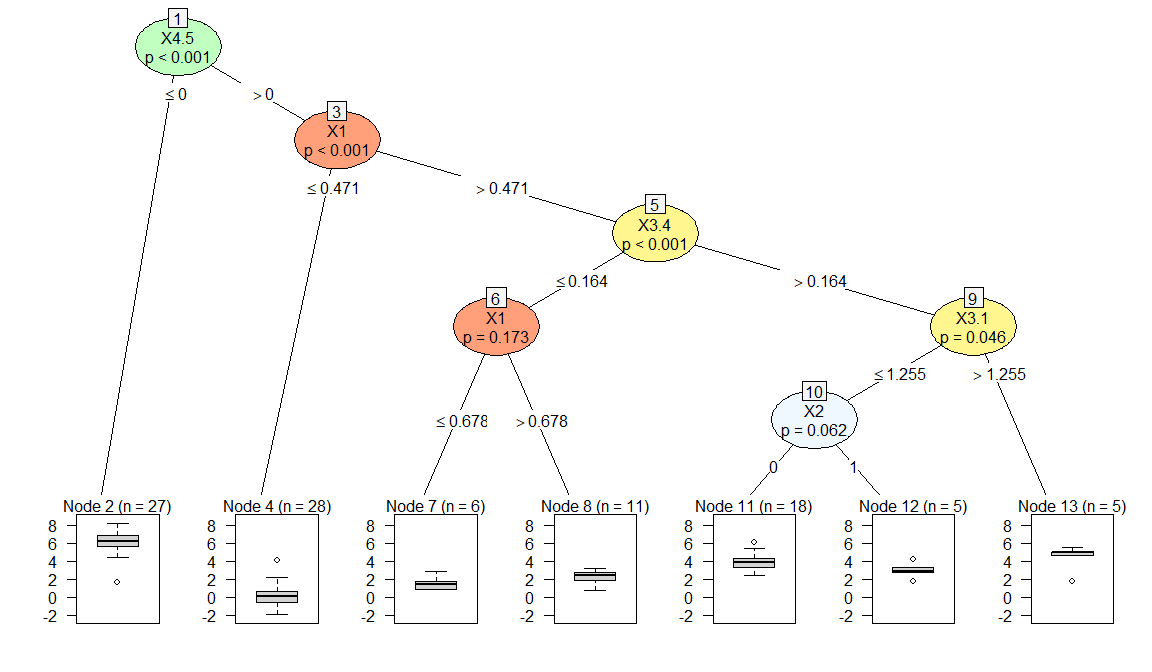We obtain a much larger tree with $$7$$ terminal nodes. Splits are made with respect to all four covariates, hence showing the full spectrum of colors for the variables’ types that are currently accepted by etree().

Another important argument is split_type, which defines the splitting method. By default it is equal to “coeff”, so we can now try to fit a tree using “cluster”.

# Fit with 'cluster'
etree_fit3 <- etree(response = resp_reg,
covariates = cov_list,
split_type = 'cluster')

# Plot
plot(etree_fit3)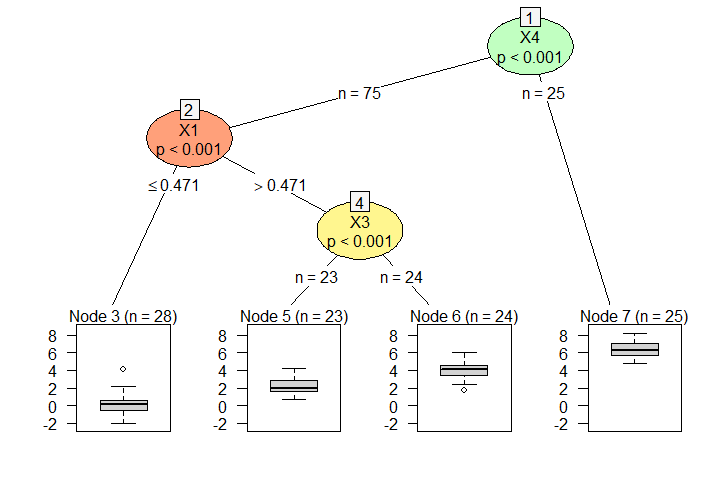In this case, we obtain a tree that is structurally equivalent and has the same hierarchy among covariates as the one produced with split_type = “coeff”. The tiny differences in the terminal nodes’ size are due to the change in the splitting method for covariates $$X_3$$ and $$X_4$$.

This suggests that the two splitting strategies produce consistent and sensible results, which are nonetheless different, so it does make sense to grow trees with both of them and compare the output.

The plot allows visualizing two differences that are specific to the case of split_type = “cluster”: the text below the square box is always the name of the splitting covariate, regardless of its nature; when the splitting covariate is structured, values on the edges represent the corresponding kid node’s size.

## Predict

Predictions are obtained in a very classical way: by calling predict() on the fitted Energy Tree.

They are based either on the fitted values - if newdata is not provided - or on the new set of covariates - if newdata is specified.

All the necessary information, such as the splitting strategy or the type of the task, is automatically retrieved from the fitted object.

# Predictions from the fitted object
pred <- predict(etree_fit)
print(pred)
#>         4         4         4         4         4         4         4         4
#> 0.2030867 0.2030867 0.2030867 0.2030867 0.2030867 0.2030867 0.2030867 0.2030867
#>         4         4         4         4         4         4         4         4
#> 0.2030867 0.2030867 0.2030867 0.2030867 0.2030867 0.2030867 0.2030867 0.2030867
#>         4         4         4         4         4         4         4         4
#> 0.2030867 0.2030867 0.2030867 0.2030867 0.2030867 0.2030867 0.2030867 0.2030867
#>         4         4         7         4         6         7         6         6
#> 0.2030867 0.2030867 3.8091424 0.2030867 1.9798255 3.8091424 1.9798255 1.9798255
#>         6         6         6         6         6         6         6         6
#> 1.9798255 1.9798255 1.9798255 1.9798255 1.9798255 1.9798255 1.9798255 1.9798255
#>         6         7         6         7         6         6         2         6
#> 1.9798255 3.8091424 1.9798255 3.8091424 1.9798255 1.9798255 6.0385608 1.9798255
#>         7         6         7         7         7         7         7         7
#> 3.8091424 1.9798255 3.8091424 3.8091424 3.8091424 3.8091424 3.8091424 3.8091424
#>         7         7         7         7         2         7         7         7
#> 3.8091424 3.8091424 3.8091424 3.8091424 6.0385608 3.8091424 3.8091424 3.8091424
#>         7         7         7         4         7         7         7         7
#> 3.8091424 3.8091424 3.8091424 0.2030867 3.8091424 3.8091424 3.8091424 3.8091424
#>         7         7         7         2         2         2         2         2
#> 3.8091424 3.8091424 3.8091424 6.0385608 6.0385608 6.0385608 6.0385608 6.0385608
#>         2         2         2         2         2         2         2         2
#> 6.0385608 6.0385608 6.0385608 6.0385608 6.0385608 6.0385608 6.0385608 6.0385608
#>         2         2         2         2         2         2         2         2
#> 6.0385608 6.0385608 6.0385608 6.0385608 6.0385608 6.0385608 6.0385608 6.0385608
#>         2         2         2         2
#> 6.0385608 6.0385608 6.0385608 6.0385608

The division into nodes obtained partitioning with Energy Trees reduce the response’s variability with respect to the original data. Indeed, the Mean Square Error between the response and the predicted values is more than $$80\%$$ smaller than that between the response and its average.

# MSE between the response and its average
mean((resp_reg - mean(resp_reg)) ^ 2)
#>  6.252811

# MSE between the response and predictions from the fitted tree
mean((resp_reg - pred) ^ 2)
#>  1.217504

To understand if this behavior is robust, we can perform the same comparison using a new set of data. We generate $$40$$ observations using the same mechanism as before, and then we use the new set of covariates to get predictions using predict() with newdata.

# New set of covariates
n_obs <- 40
x1n <- c(runif(n_obs / 4, min = 0, max = 0.55),
runif(n_obs / 4 * 3, min = 0.45, max = 1))
x2n <- factor(c(rbinom(n_obs / 4, 1, 0.1),
rbinom(n_obs / 4, 1, 0.9),
rbinom(n_obs / 2, 1, 0.1)))
x3n <- c(fda.usc::rproc2fdata(n_obs / 2, seq(0, 1, len = 100), sigma = 1),
fda.usc::rproc2fdata(n_obs / 4, seq(0, 1, len = 100), sigma = 1,
mu = rep(1, 100)),
fda.usc::rproc2fdata(n_obs / 4, seq(0, 1, len = 100), sigma = 1))
x4n <- c(lapply(1:(n_obs / 4 * 3), function(j) igraph::sample_gnp(100, 0.1)),
lapply(1:(n_obs / 4), function(j) igraph::sample_gnp(100, 0.9)))
new_cov_list <- list(X1 = x1n, X2 = x2n, X3 = x3n, X4 = x4n)

# New response
new_resp_reg <- c(rnorm(n_obs / 4, mean = 0, sd = 1),
rnorm(n_obs / 4, mean = 2, sd = 1),
rnorm(n_obs / 4, mean = 4, sd = 1),
rnorm(n_obs / 4, mean = 6, sd = 1))

# Predictions
new_pred <- predict(etree_fit,
newdata = new_cov_list)
print(new_pred)
#>         4         4         6         6         4         4         4         4
#> 0.2030867 0.2030867 1.9798255 1.9798255 0.2030867 0.2030867 0.2030867 0.2030867
#>         4         4         7         6         6         7         6         7
#> 0.2030867 0.2030867 3.8091424 1.9798255 1.9798255 3.8091424 1.9798255 3.8091424
#>         7         6         7         6         7         7         7         7
#> 3.8091424 1.9798255 3.8091424 1.9798255 3.8091424 3.8091424 3.8091424 3.8091424
#>         2         7         6         7         7         7         2         2
#> 6.0385608 3.8091424 1.9798255 3.8091424 3.8091424 3.8091424 6.0385608 6.0385608
#>         2         2         2         2         2         2         2         2
#> 6.0385608 6.0385608 6.0385608 6.0385608 6.0385608 6.0385608 6.0385608 6.0385608

The comparison in terms of MSE is as follows.

# MSE between the new response and its average
mean((new_resp_reg - mean(new_resp_reg)) ^ 2)
#>  5.667461

# MSE between the new response and predictions with the new set of covariates
mean((new_resp_reg - new_pred) ^ 2)
#>  0.9968288

Hence, the behavior is confirmed also with a new set of data.

# Classification

The second example is classification. The set of covariates is the same as before, i.e., cov_list, while the response variable is resp_cls. Also in this case, a quick fit can be obtained by calling etree() and specifying the response and the set of covariates.

# Quick fit
etree_fit <- etree(response = resp_cls,
covariates = cov_list)

# Plot
plot(etree_fit)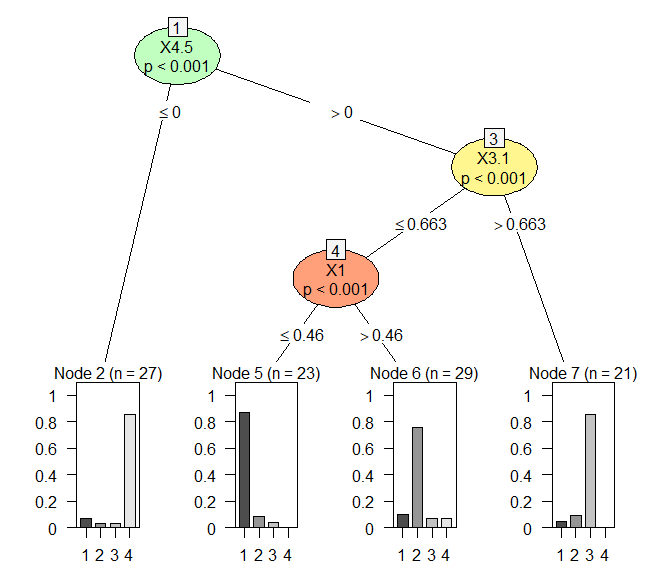The only difference with respect to plots for regression tasks is the content of the terminal panels: in the case of classification, they show the distribution of the response variable for each node using bar plots.

In the specific example, the structure and size of the tree is coherent with the true data partition made of four groups, and the levels are almost perfectly recognized.

For classification, we will not see any change for the default values of the optional parameters since they work in the same way as for regression.

Also printing and the other utilities work in the same way we saw before.

Predictions are obtained as for regression, but we cover this part also for classification for the sake of completeness.

# Predictions from the fitted object
pred <- predict(etree_fit)
print(pred)
#> 5 5 5 5 5 5 5 5 5 5 5 5 5 5 5 5 5 5 5 6 6 5 5 5 5 6 6 6 6 6 6 6 6 6 6 6 6 6 6 6
#> 1 1 1 1 1 1 1 1 1 1 1 1 1 1 1 1 1 1 1 2 2 1 1 1 1 2 2 2 2 2 2 2 2 2 2 2 2 2 2 2
#> 6 6 7 6 6 6 2 6 6 6 7 7 7 6 7 7 7 7 7 7 2 6 7 7 7 7 7 7 7 6 6 7 7 7 7 2 2 2 2 2
#> 2 2 3 2 2 2 4 2 2 2 3 3 3 2 3 3 3 3 3 3 4 2 3 3 3 3 3 3 3 2 2 3 3 3 3 4 4 4 4 4
#> 2 2 2 2 2 2 2 2 2 2 2 2 2 2 2 2 2 2 2 2
#> 4 4 4 4 4 4 4 4 4 4 4 4 4 4 4 4 4 4 4 4
#> Levels: 1 2 3 4

We can use the confusion matrix between the response and the predicted values, and a scalar measure such as the misclassification error, to evaluate the predictive ability of the fitted tree.

# Confusion matrix between the response and predictions from the fitted tree
table(pred, resp_cls, dnn = c('Predicted', 'True'))
#>          True
#> Predicted  1  2  3  4
#>         1 20  2  1  0
#>         2  3 22  2  2
#>         3  1  2 18  0
#>         4  2  1  1 23

# Misclassification error for predictions from the fitted tree
sum(pred != resp_cls) / length(resp_cls)
#>  0.17

The tree produces sensible predictions for fitted data. We can verify how it generalizes to a new set of covariates. We can use the one generated for regression, but we still need to draw a new response variable for classification.

# New response
new_resp_cls <- factor(c(sample(x = 1:4, size = n_obs / 4, replace = TRUE,
prob = c(0.85, 0.05, 0.05, 0.05)),
sample(x = 1:4, size = n_obs / 4, replace = TRUE,
prob = c(0.05, 0.85, 0.05, 0.05)),
sample(x = 1:4, size = n_obs / 4, replace = TRUE,
prob = c(0.05, 0.05, 0.85, 0.05)),
sample(x = 1:4, size = n_obs / 4, replace = TRUE,
prob = c(0.05, 0.05, 0.05, 0.85))))

# Predictions
new_pred <- predict(etree_fit,
newdata = new_cov_list)
print(new_pred)
#> 5 5 6 6 5 5 5 5 5 5 6 6 6 6 7 6 6 6 6 6 7 6 6 7 2 6 7 7 7 6 2 2 2 2 2 2 2 2 2 2
#> 1 1 2 2 1 1 1 1 1 1 2 2 2 2 3 2 2 2 2 2 3 2 2 3 4 2 3 3 3 2 4 4 4 4 4 4 4 4 4 4
#> Levels: 1 2 3 4

# Confusion matrix between the new response and predictions from the fitted tree
table(new_pred, new_resp_cls, dnn = c('Predicted', 'True'))
#>          True
#> Predicted 1 2 3 4
#>         1 7 0 1 0
#>         2 3 7 4 1
#>         3 0 1 5 0
#>         4 1 0 1 9

# Misclassification error for predictions on the new set of covariates
sum(new_pred != new_resp_cls) / length(new_resp_cls)
#>  0.3

Taking into account that the set of covariates and the response variable have been generated using a fuzzy scheme both for fitting and testing data, predictions using the new set can be considered satisfactory.